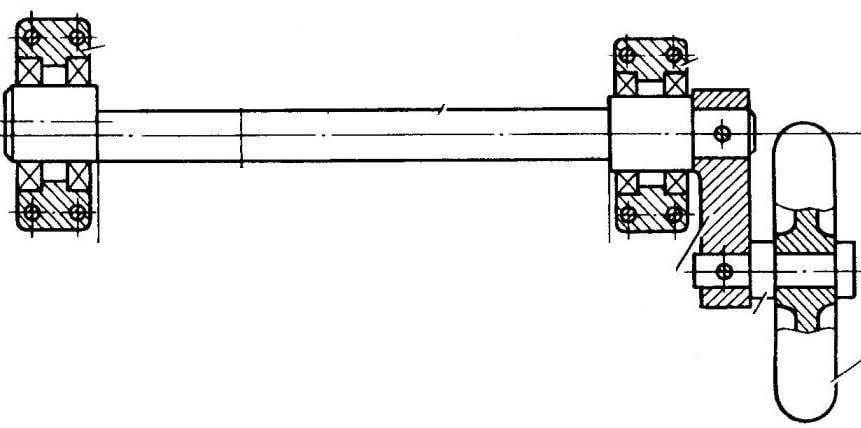# TORSION ECCENTRIC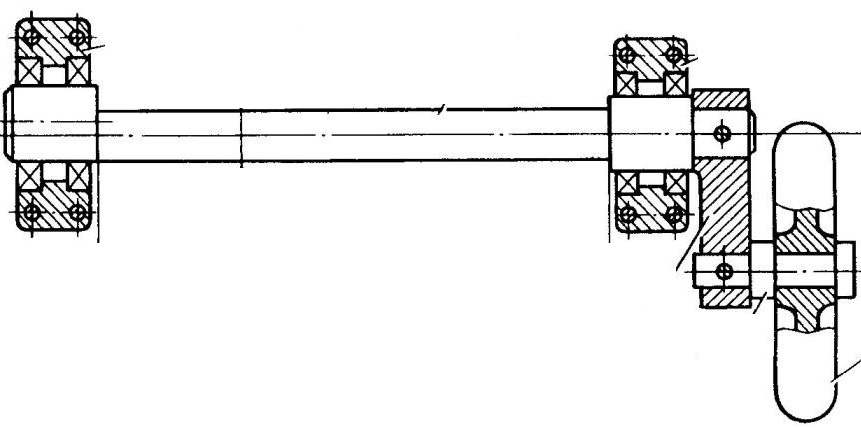The presented design can be used in homemade vehicles (e.g., cars or velomobiles) as a torsion suspension of the wheels. The basis of her supposed domestic the invention.with. No. 184564, кл47а, 17 “torsion Spring”.

The main detail of the structure is elastic element in the form of ball bearings installed in the shaft with two eccentric spaced relative to each other reference journals. The eccentricity e, the length l and the diameter d of the shaft completely determine its stiffness and strength characteristics.

How does the suspension?
The wheel rotates freely on its axis, fixed in the lever parallel to the longitudinal axis of the eccentric shaft. Assuming that the lever and the longitudinal axis located in the same horizontal plane, the load on the suspension will be expressed as vertical (perpendicular to the plane of the drawing) efforts Q of the wheel and the torque MD is equal to the product of the magnitude of this effort on the lever arm R. the Very force Q, equal to about a fourth of the weight of the vehicle (if it is a four-wheeled), perceived the bearing supports, while the share of eccentric shaft has a torque value MD.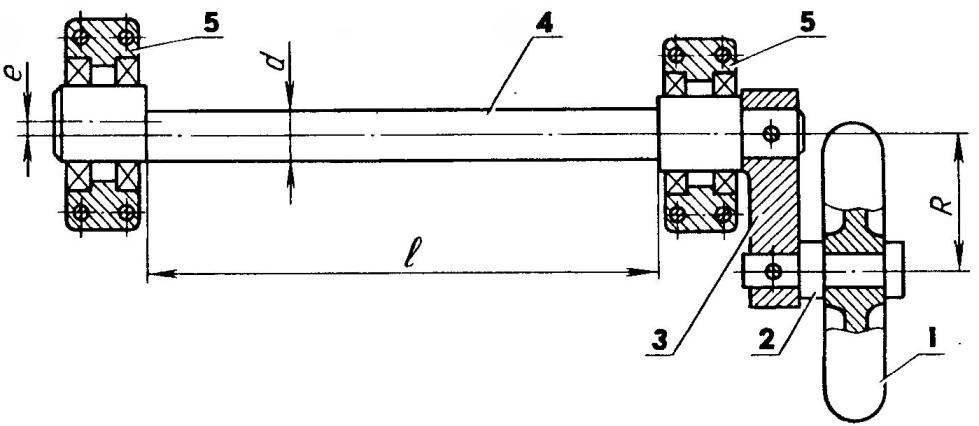Torque spring (eccentric shaft) in the role of the elastic element of the torsion suspension of the wheels:

1 — wheel; 2 — axle; 3 — lever; 4 — the eccentric shaft; 5 — bearing supports.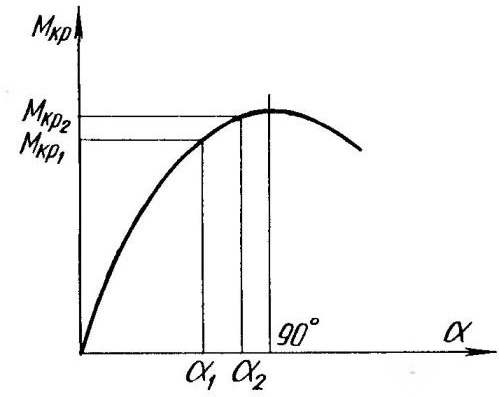Zhestkosty characteristics of the eccentric shaft.

As a result, it was found in the experiments, except for the usual torsion bar torsion, and bending there, considerably exceeding the first, which leads to rotation of the shaft at an angle, and balances the action of torque.

Properly chosen geometrical parameters of the eccentric shaft ensure a high elasticity of the suspension in a wide range of variations in torque due to the nonlinear (sinusoidal) stiffness in shoulde-ing characteristics of the elastic element.
From this it follows that the sloping portion of the characteristic between the maximum angular deformation of the eccentric shaft a, and A2 close to 90°, corresponds to a slight change in the magnitude of the actual load — torque.

This is one of the advantages of the described suspension over conventional twisted torsion, zhestkosty feature which is straightforward and has no flat areas.

The calculation of the stiffness of the torsion bar with eccentric shaft can be made according to the following formula: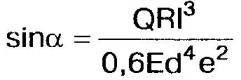where a is the largest angle of the elastic twisting of the eccentric shaft, grad.; Q is the force acting on the wheel, kg; R is the shoulder of this force (the lever length), cm; I — the calculated shaft length, cm; E is modulus of elasticity, kg/cm2; d — diameter of bending of the shaft, cm; e — the eccentricity of the shaft, see

This formula indicates a sinusoidal dependence of the angle of the crank shaft from the existing force Q.

The calculation of the strength of the shaft can be performed according to the formula: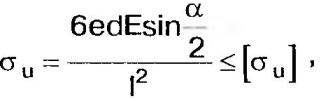where AI — the bending stresses of the shaft, kg/cm2; [b] — permissible bending stresses, kg/cm2.

Selecting geometric parameters of the shaft and the magnitude of the permissible elastic deformation, should provide constructive work of the torsion within the design limits, for example, by setting the lever of limit stops made of rubber or plastic.

A. EGISHYANTS,
Obninsk,
The Kaluga region.